Скачать презентацию Spatial Range Querying for Gaussian-Based Imprecise Query Objects

e2d4a1c42fea846653d4621f4ccae310.ppt

• Количество слайдов: 37Spatial Range Querying for Gaussian-Based Imprecise Query Objects Yoshiharu Ishikawa, Yuichi Iijima Nagoya University Jeffrey Xu Yu The Chinese University of Hong Kong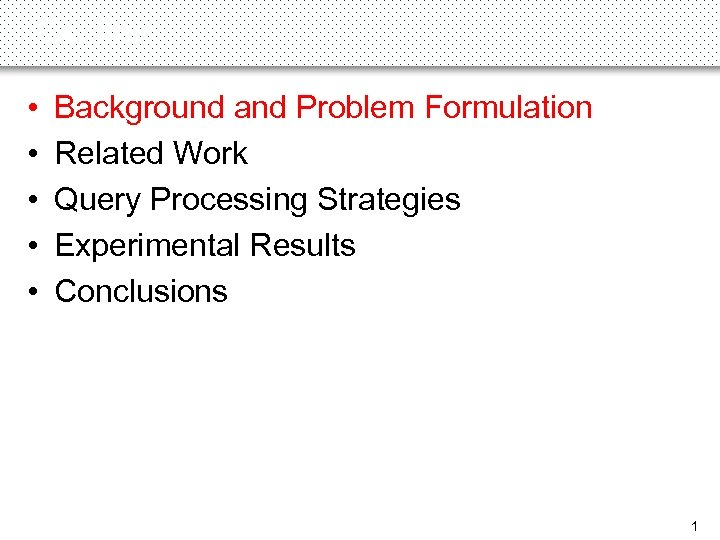Outline • • • Background and Problem Formulation Related Work Query Processing Strategies Experimental Results Conclusions 1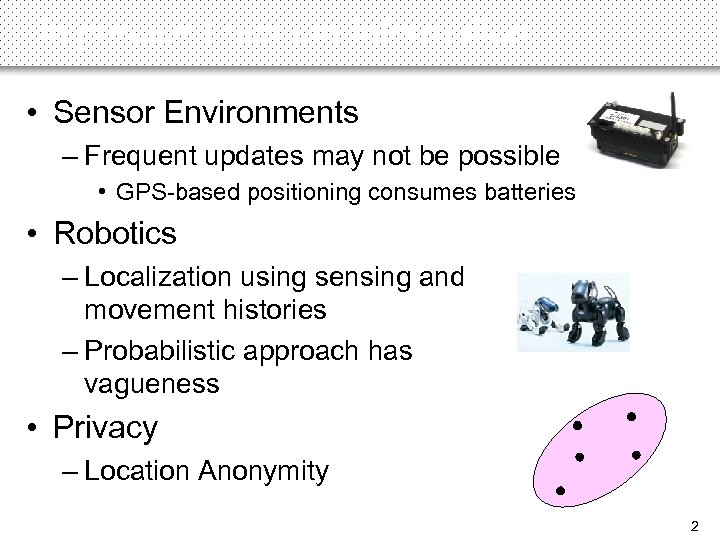Imprecise Location Information • Sensor Environments – Frequent updates may not be possible • GPS-based positioning consumes batteries • Robotics – Localization using sensing and movement histories – Probabilistic approach has vagueness • Privacy – Location Anonymity 2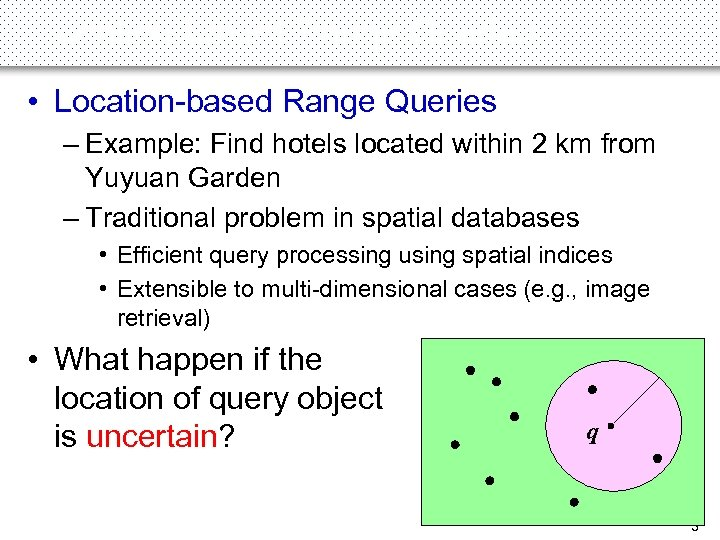Location-based Range Queries • Location-based Range Queries – Example: Find hotels located within 2 km from Yuyuan Garden – Traditional problem in spatial databases • Efficient query processing using spatial indices • Extensible to multi-dimensional cases (e. g. , image retrieval) • What happen if the location of query object is uncertain? q 3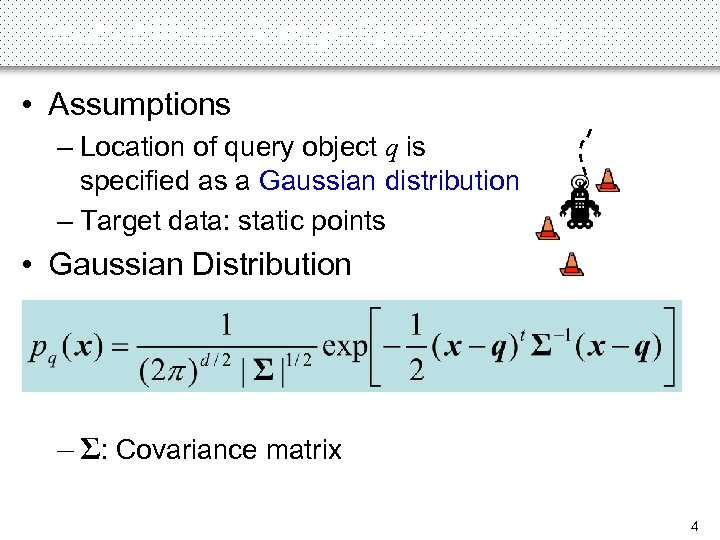Probabilistic Range Query (PRQ) (1) • Assumptions – Location of query object q is specified as a Gaussian distribution – Target data: static points • Gaussian Distribution – Σ: Covariance matrix 4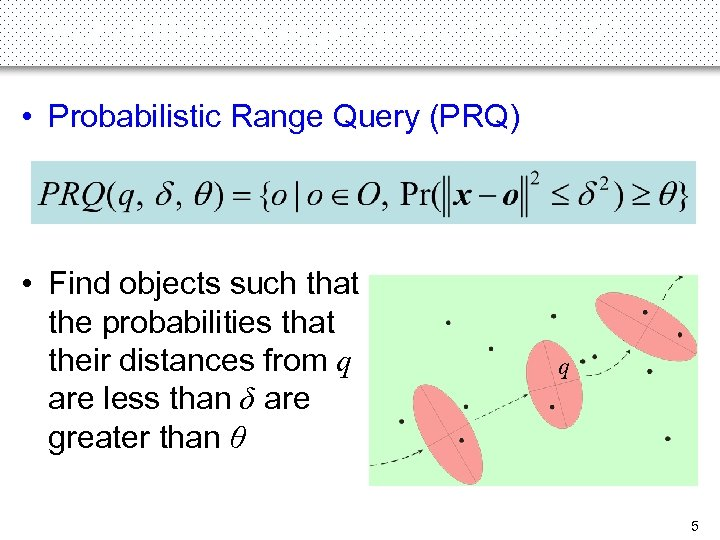Probabilistic Range Query (PRQ) (2) • Probabilistic Range Query (PRQ) • Find objects such that the probabilities that their distances from q are less than δ are greater than θ q 5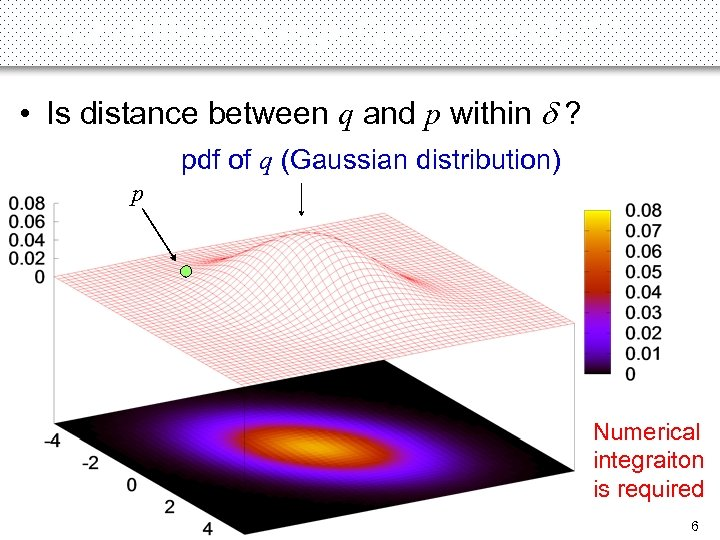Probabilistic Range Query (PRQ) (3) • Is distance between q and p within d ? pdf of q (Gaussian distribution) p Numerical integraiton is required 6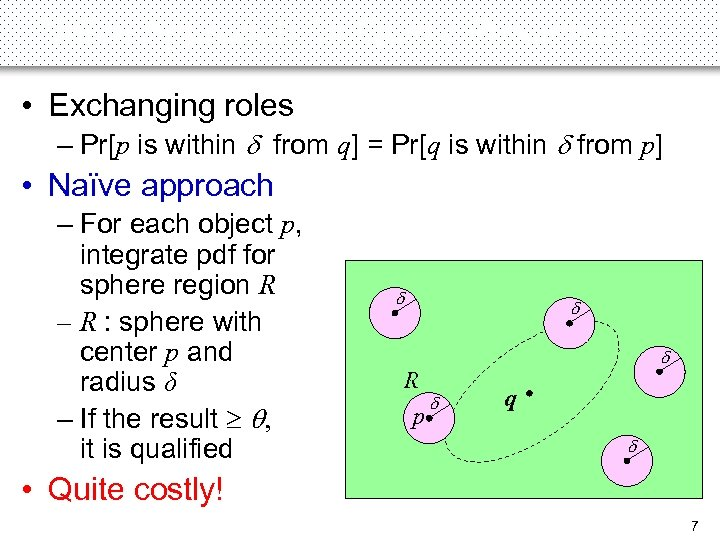Naïve Approach for Query Processing • Exchanging roles – Pr[p is within d from q] = Pr[q is within d from p] • Naïve approach – For each object p, integrate pdf for sphere region R – R : sphere with center p and radius δ – If the result , it is qualified d d R d p d q d • Quite costly! 7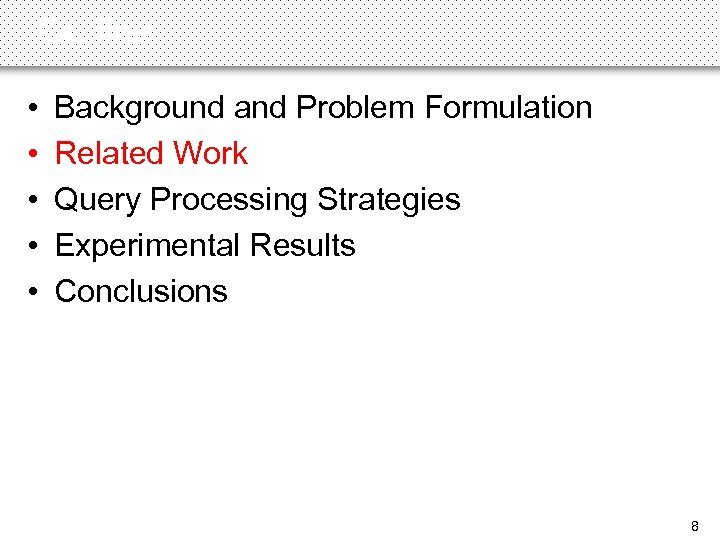Outline • • • Background and Problem Formulation Related Work Query Processing Strategies Experimental Results Conclusions 8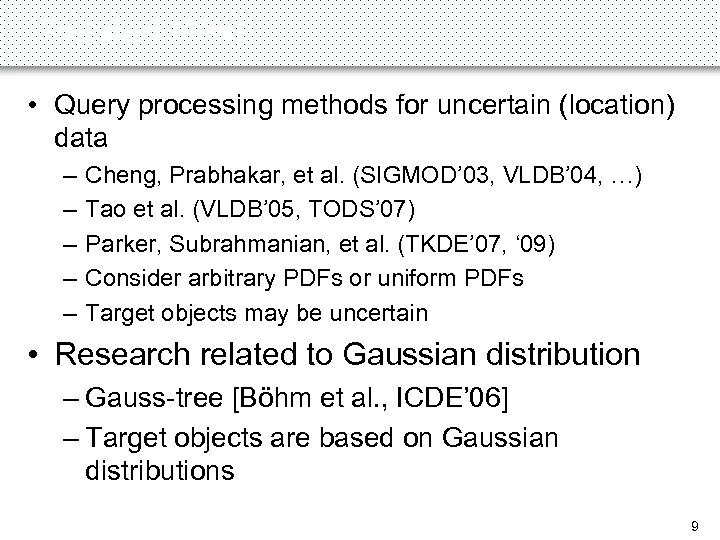Related Work • Query processing methods for uncertain (location) data – – – Cheng, Prabhakar, et al. (SIGMOD’ 03, VLDB’ 04, …) Tao et al. (VLDB’ 05, TODS’ 07) Parker, Subrahmanian, et al. (TKDE’ 07, ‘ 09) Consider arbitrary PDFs or uniform PDFs Target objects may be uncertain • Research related to Gaussian distribution – Gauss-tree [Böhm et al. , ICDE’ 06] – Target objects are based on Gaussian distributions 9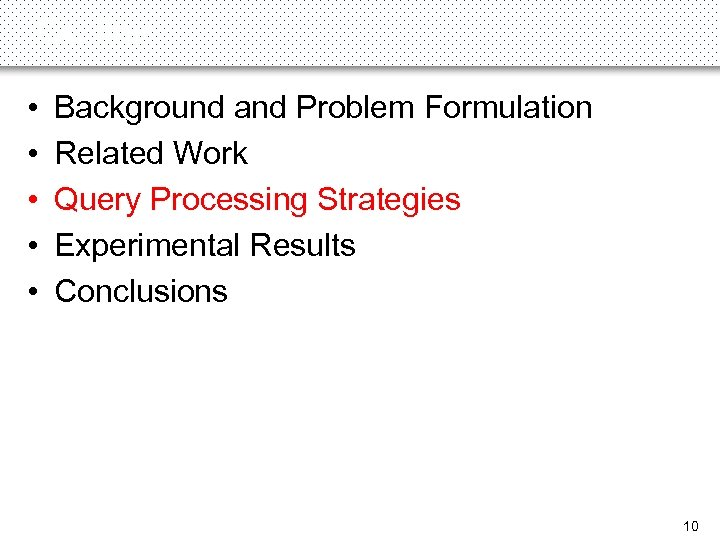Outline • • • Background and Problem Formulation Related Work Query Processing Strategies Experimental Results Conclusions 10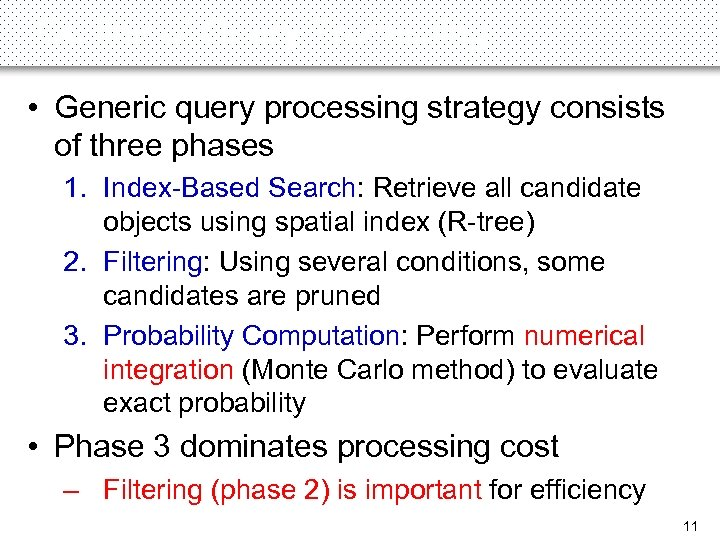Outline of Query Processing • Generic query processing strategy consists of three phases 1. Index-Based Search: Retrieve all candidate objects using spatial index (R-tree) 2. Filtering: Using several conditions, some candidates are pruned 3. Probability Computation: Perform numerical integration (Monte Carlo method) to evaluate exact probability • Phase 3 dominates processing cost – Filtering (phase 2) is important for efficiency 11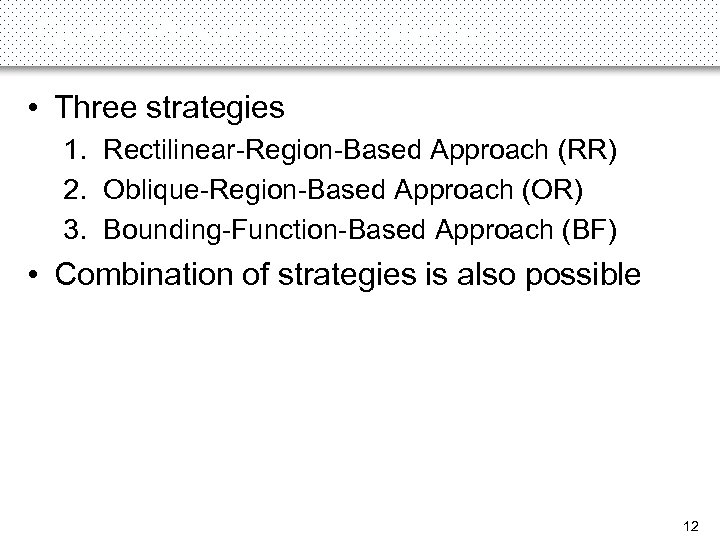Query Processing Strategies • Three strategies 1. Rectilinear-Region-Based Approach (RR) 2. Oblique-Region-Based Approach (OR) 3. Bounding-Function-Based Approach (BF) • Combination of strategies is also possible 12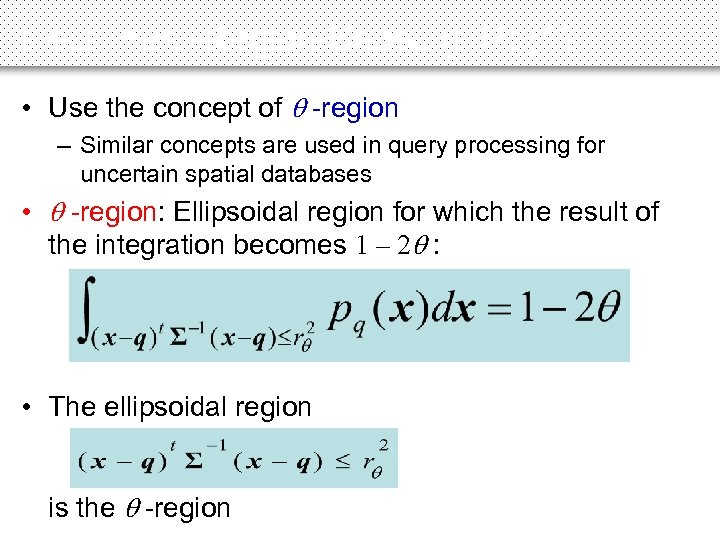Rectilinear-Region-Based (RR) (1) • Use the concept of -region – Similar concepts are used in query processing for uncertain spatial databases • -region: Ellipsoidal region for which the result of the integration becomes 1 – 2 : • The ellipsoidal region is the -region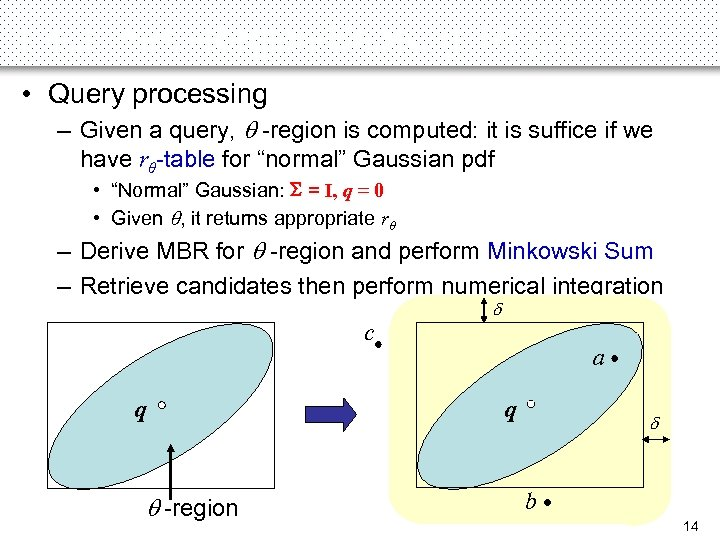Rectilinear-Region-Based (RR) (2) • Query processing – Given a query, -region is computed: it is suffice if we have rθ-table for “normal” Gaussian pdf • “Normal” Gaussian: S = I, q = 0 • Given , it returns appropriate rθ – Derive MBR for -region and perform Minkowski Sum – Retrieve candidates then perform numerical integration c q -region d a q d b 14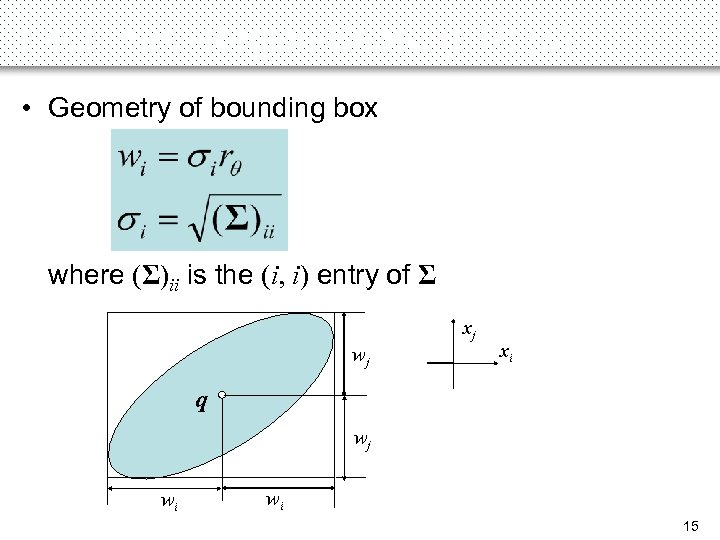Rectilinear-Region-Based (RR) (3) • Geometry of bounding box where (Σ)ii is the (i, i) entry of Σ xj wj xi q wj wi wi 15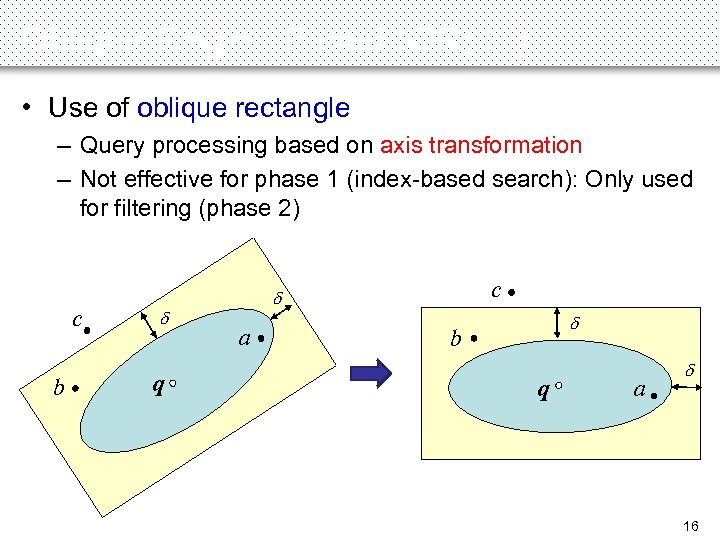Oblique-Region-Based (OR) (1) • Use of oblique rectangle – Query processing based on axis transformation – Not effective for phase 1 (index-based search): Only used for filtering (phase 2) c b d q c d a d b q a d 16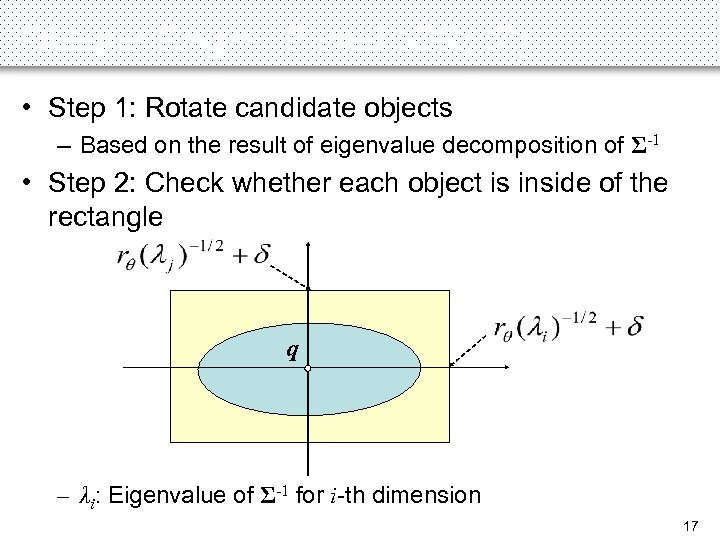Oblique-Region-Based (OR) (2) • Step 1: Rotate candidate objects – Based on the result of eigenvalue decomposition of Σ-1 • Step 2: Check whether each object is inside of the rectangle q – λi: Eigenvalue of Σ-1 for i-th dimension 17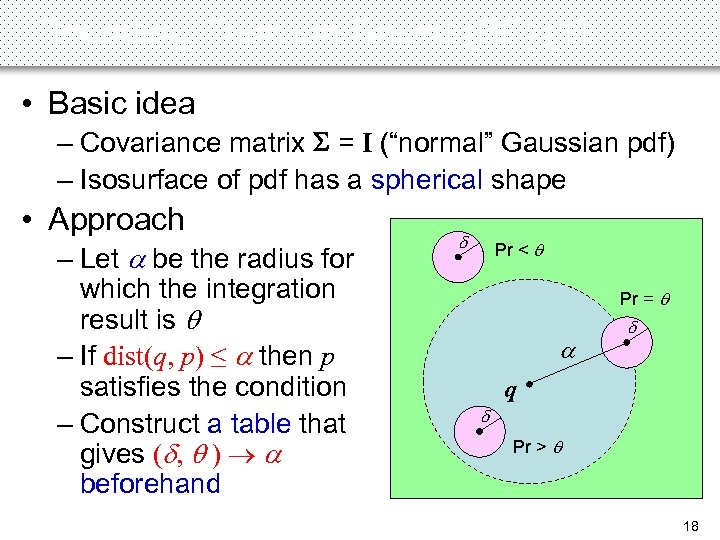Bounding-Function-Based (BF) (1) • Basic idea – Covariance matrix S = I (“normal” Gaussian pdf) – Isosurface of pdf has a spherical shape • Approach – Let a be the radius for which the integration result is – If dist(q, p) ≤ a then p satisfies the condition – Construct a table that gives (d, ) a beforehand d Pr < Pr = a d q d Pr > 18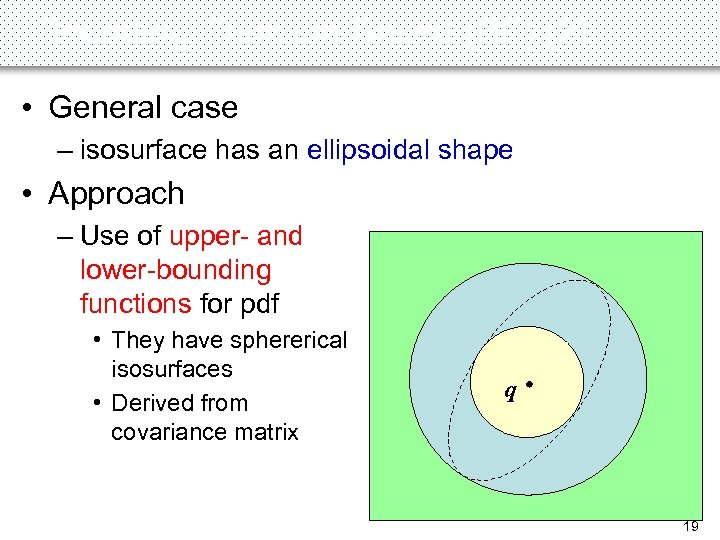Bounding-Function-Based (BF) (2) • General case – isosurface has an ellipsoidal shape • Approach – Use of upper- and lower-bounding functions for pdf • They have sphererical isosurfaces • Derived from covariance matrix q 19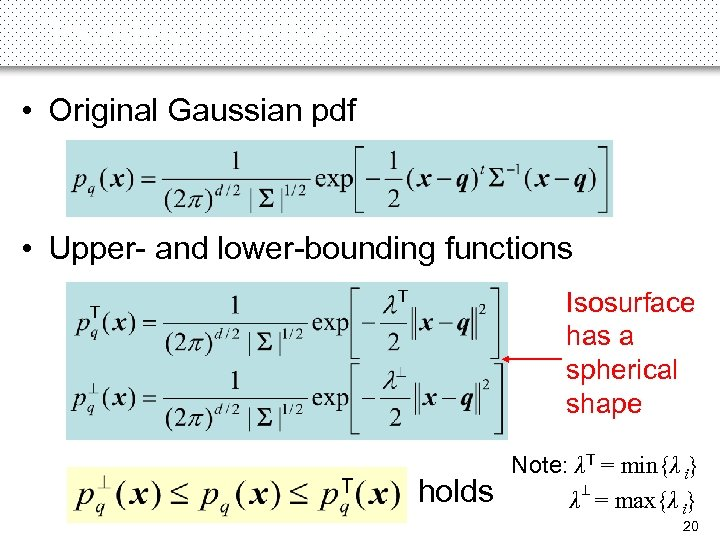Bounding Functions • Original Gaussian pdf T T • Upper- and lower-bounding functions Isosurface has a spherical shape T T holds Note: λT = min{λ i} λ = max{λ i} T T 20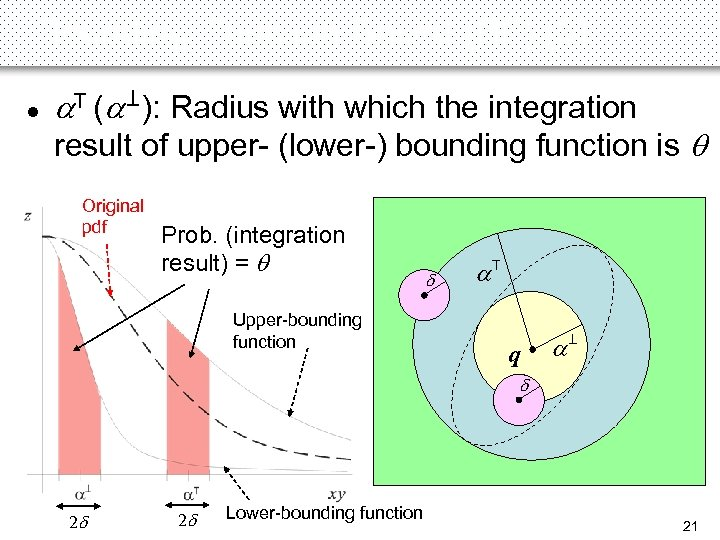Bounding-Function-Based (BF) (3) a. T (a ): Radius with which the integration T result of upper- (lower-) bounding function is Original pdf Prob. (integration result) = Upper-bounding function d a. T q a T l d 2 d 2 d Lower-bounding function 21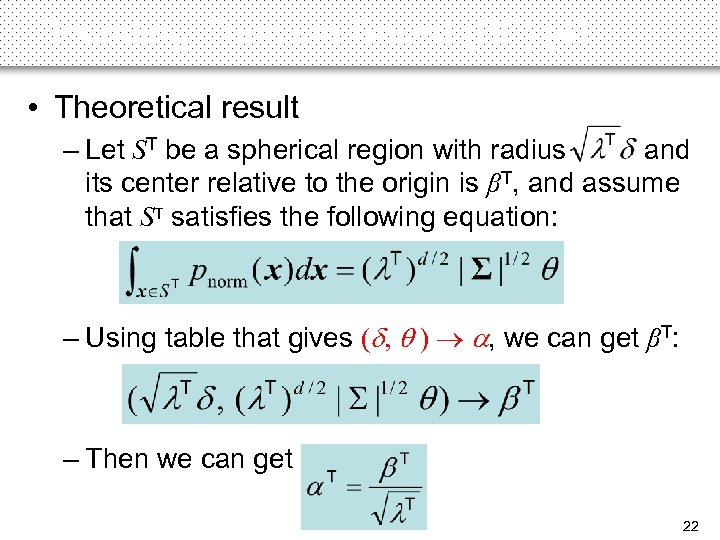Bounding-Function-Based (BF) (4) • Theoretical result – Let ST be a spherical region with radius and its center relative to the origin is βT, and assume that ST satisfies the following equation: – Using table that gives (d, ) a, we can get βT: – Then we can get 22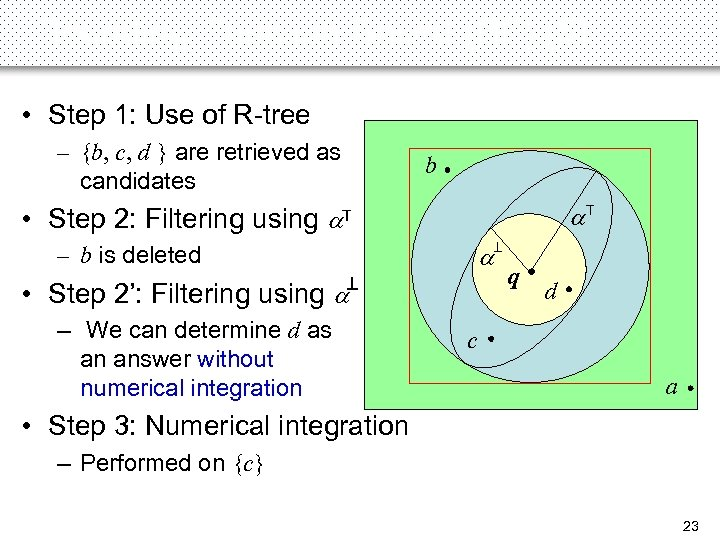Bounding-Function-Based (BF) (5) • Step 1: Use of R-tree – {b, c, d } are retrieved as candidates a. T T a – b is deleted T • Step 2’: Filtering using a – We can determine d as an answer without numerical integration T • Step 2: Filtering using a b q d c a • Step 3: Numerical integration – Performed on {c} 23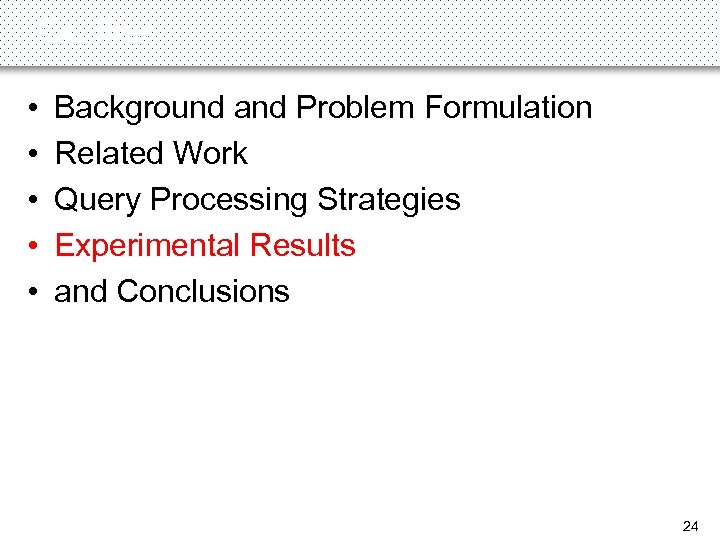Outline • • • Background and Problem Formulation Related Work Query Processing Strategies Experimental Results and Conclusions 24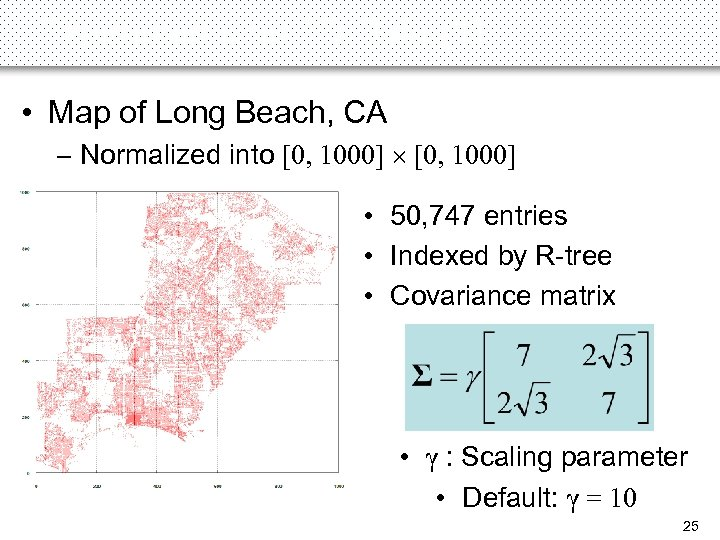Experiments on 2 D Data (1) • Map of Long Beach, CA – Normalized into [0, 1000] • 50, 747 entries • Indexed by R-tree • Covariance matrix • γ : Scaling parameter • Default: γ = 10 25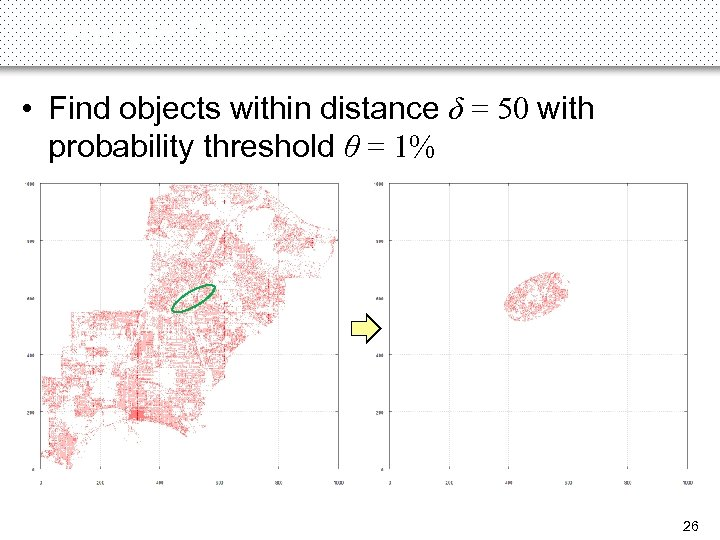Example Query • Find objects within distance δ = 50 with probability threshold θ = 1% 26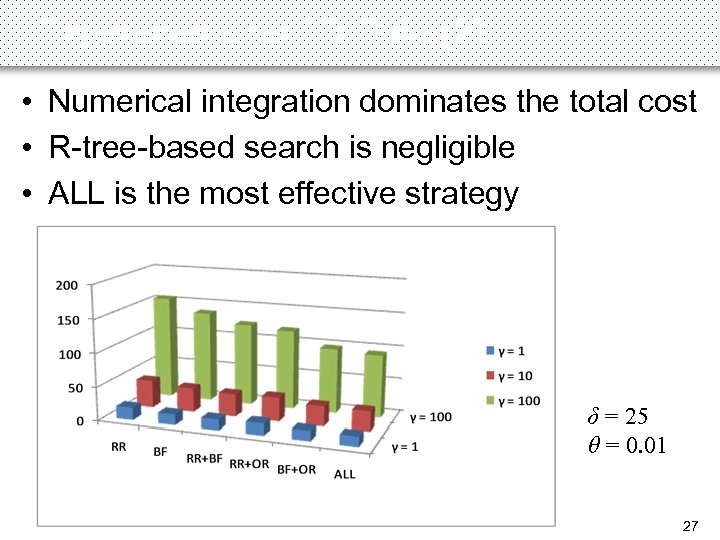Experiments on 2 D Data (2) • Numerical integration dominates the total cost • R-tree-based search is negligible • ALL is the most effective strategy δ = 25 θ = 0. 01 27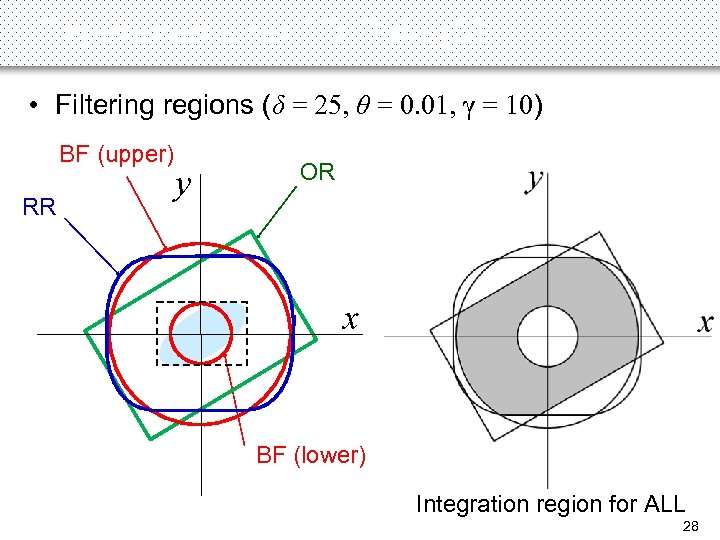Experiments on 2 D Data (3) • Filtering regions (δ = 25, θ = 0. 01, γ = 10) BF (upper) RR y OR x BF (lower) I Integration region for ALL 28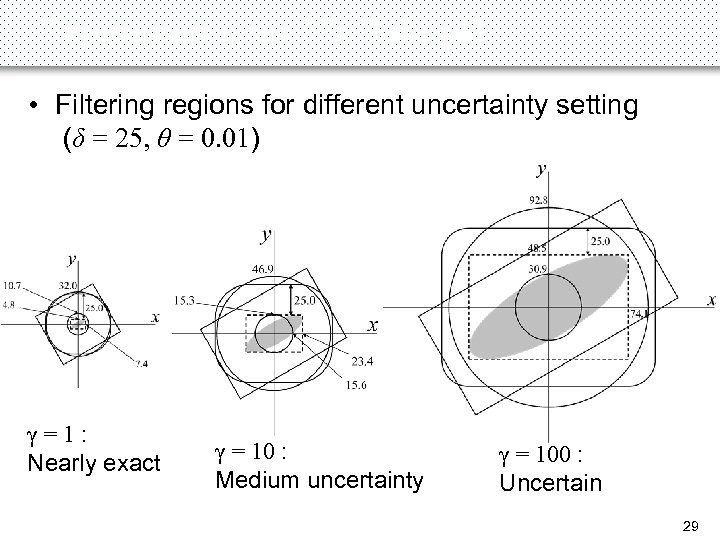Experiments on 2 D Data (4) • Filtering regions for different uncertainty setting (δ = 25, θ = 0. 01) γ=1: Nearly exact γ = 10 : Medium uncertainty γ = 100 : Uncertain 29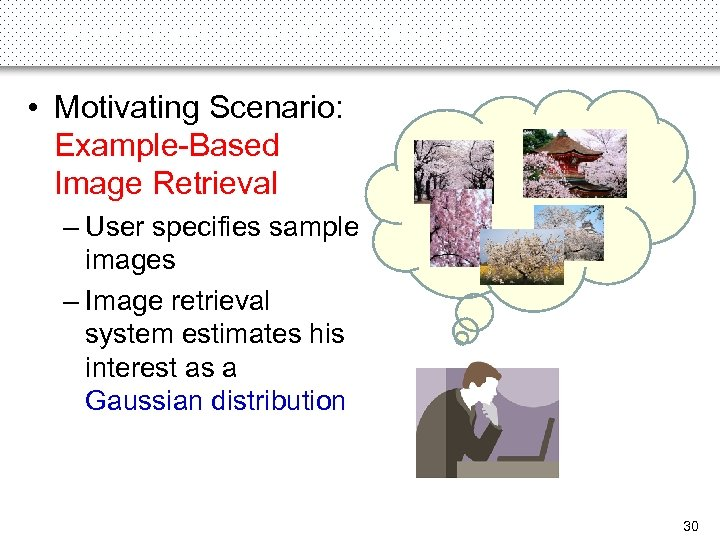Experiments on 9 D Data (1) • Motivating Scenario: Example-Based Image Retrieval – User specifies sample images – Image retrieval system estimates his interest as a Gaussian distribution 30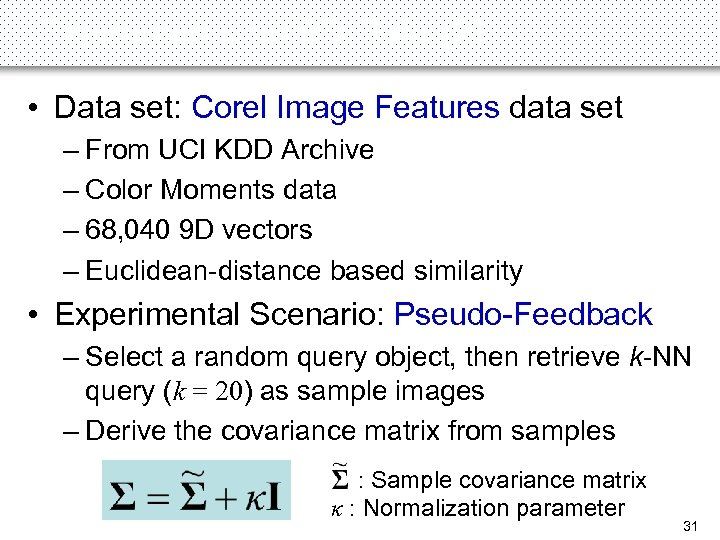Experiments on 9 D Data (2) • Data set: Corel Image Features data set – From UCI KDD Archive – Color Moments data – 68, 040 9 D vectors – Euclidean-distance based similarity • Experimental Scenario: Pseudo-Feedback – Select a random query object, then retrieve k-NN query (k = 20) as sample images – Derive the covariance matrix from samples : Sample covariance matrix κ : Normalization parameter 31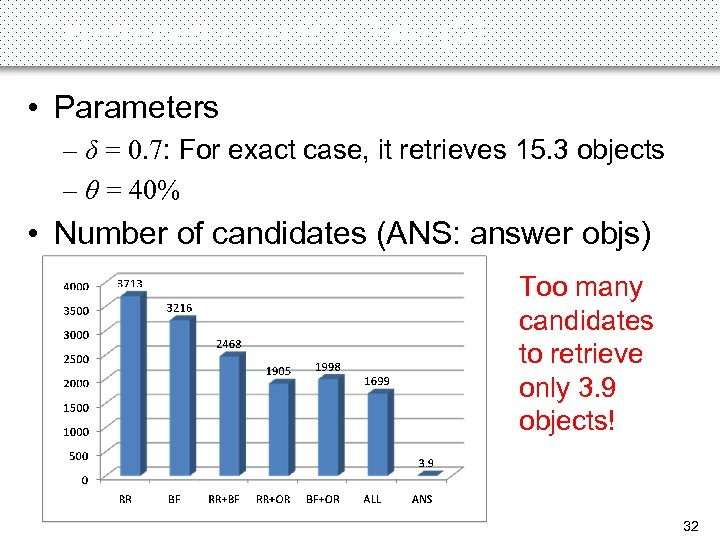Experiments on 9 D Data (3) • Parameters – δ = 0. 7: For exact case, it retrieves 15. 3 objects – θ = 40% • Number of candidates (ANS: answer objs) Too many candidates to retrieve only 3. 9 objects! 32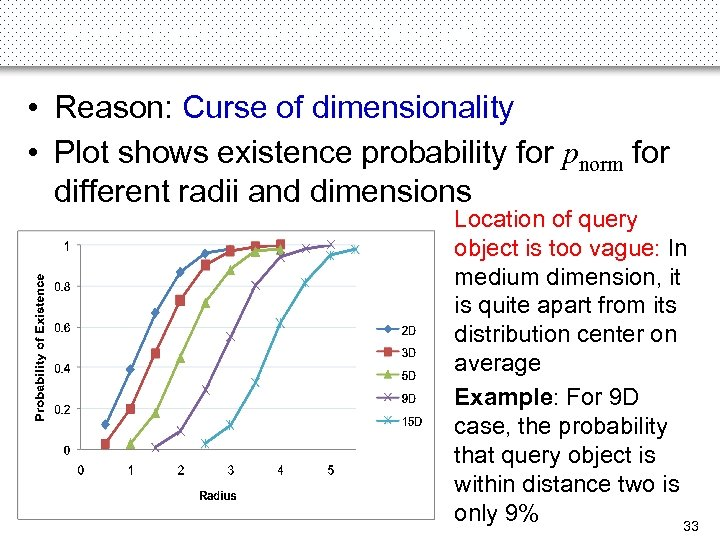Experiments on 9 D Data (4) • Reason: Curse of dimensionality • Plot shows existence probability for pnorm for different radii and dimensions Location of query object is too vague: In medium dimension, it is quite apart from its distribution center on average Example: For 9 D case, the probability that query object is within distance two is only 9% 33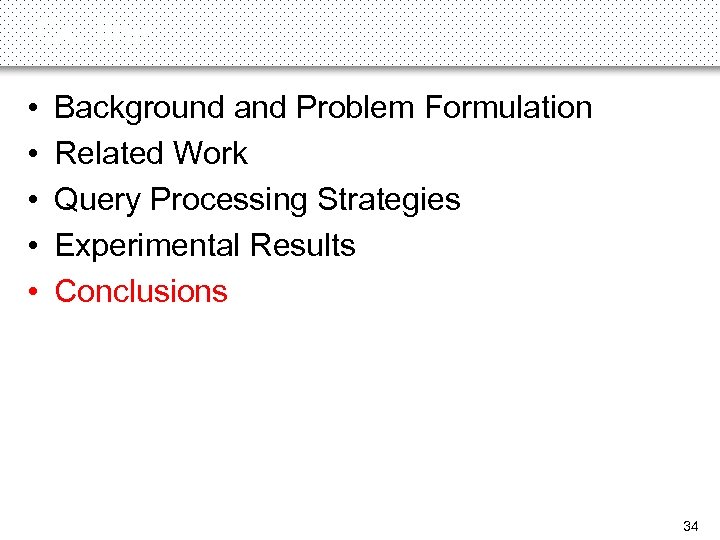Outline • • • Background and Problem Formulation Related Work Query Processing Strategies Experimental Results Conclusions 34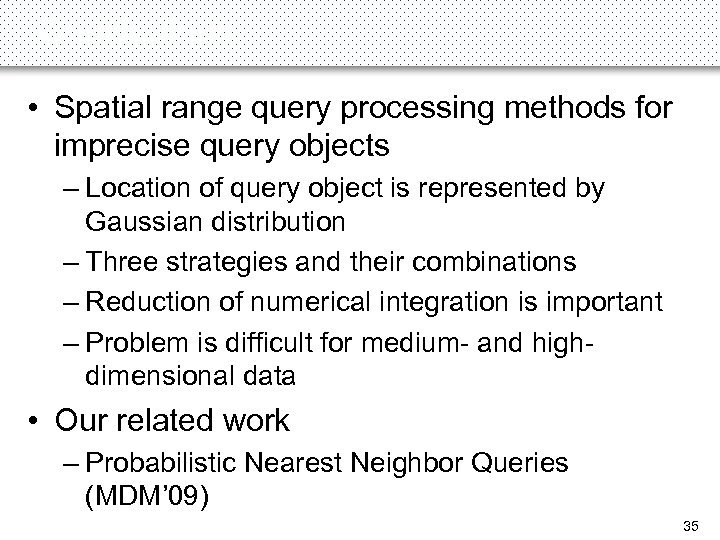Conclusions • Spatial range query processing methods for imprecise query objects – Location of query object is represented by Gaussian distribution – Three strategies and their combinations – Reduction of numerical integration is important – Problem is difficult for medium- and highdimensional data • Our related work – Probabilistic Nearest Neighbor Queries (MDM’ 09) 35Spatial Range Querying for Gaussian-Based Imprecise Query Objects Yoshiharu Ishikawa, Yuichi Iijima Nagoya University Jeffrey Xu Yu The Chinese University of Hong Kong